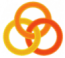General
 Course Home Page ESSLLI 2004Material
Lecture 1
 Slides Practical Session
Lecture 2
 Slides Practical Session
Lecture 3
 Slides Practical Session
Lecture 4
 Slides Practical Session
Lecture 5
 Slides Practical Session

# Working with append

Define a predicate `prefix(?Prefix,?List)` which succeeds if `Prefix` is a list which is a prefix of the list `List`. For example, `[a,b,c]` is a prefix of the list `[a,b,c,d,e]`.
Hint

Define a predicate `suffix(?Suffix,?List)` which suceeds if `Suffix` is a list which is a suffix of the list `List`. For example, `[d,e]` is a suffix of the list `[a,b,c,d,e]`.
Hint

Define a predicate `sublist(+SubL,+L)` which succeeds if `SubL` is a sublist of the list `L`. For example, `[c,d]` or `[c,d,e]` are sublists of the list `[a,b,c,d,e]`
Hint